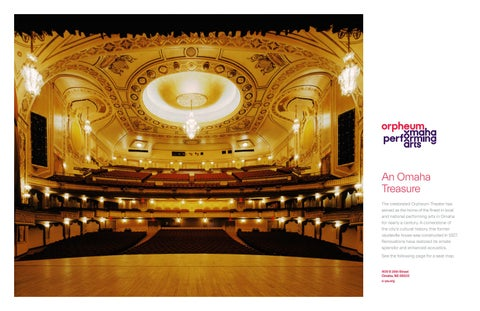# Orpheum Theater Seat MapAn Omaha Treasure The celebrated Orpheum Theater has served as the home of the finest in local and national performing arts in Omaha for nearly a century. A cornerstone of the city’s cultural history, this former vaudeville house was constructed in 1927. Renovations have restored its ornate splendor and enhanced acoustics. See the following page for a seat map.

409 S 16th Street Omaha, NE 68102 o-pa.org

Seat Map GALLERY S R Q P O N M L K

S R Q P O N M L K

S R Q P O N M L K

S R Q P O N M L K

BALCONY J H G F E D C B A

J H G F E D C B A

J H G F E D C B A

J H G F E D C B A

J H G F E D C B A

GRAND TIER D C B A

D C B A

D C B A

D C B A

LOGE

O N M L K J H G F E D C B A

T S R Q P

(P) (P) (P)

(P) (P)

(P) (P)

(P) (P) (P) (P)

(P) (P) (P) (P)

T S R Q P

T S R Q P

(P) (P)

(P) (P)

ORCHESTRA

BOXES

(W)

(C)

(C)

(C)

(C) (W)

(W)

(W) (C)

(C)

(W) (W)

(C)

(W)

(C)

(T)

(T)

(T)

(T) (T) (P) (P)

(P) (P) (T, P)

(P)

(W)

(C)

(C)

(W)

(W)

(T) (C)

Z Y X W V U T S R Q P N M L K J H G F E D C B A

(T)

BOXES

(T)

(T)

(T)

(T)

(T)

Z Y X W V U T S R Q P N M L K J H G F E D C B A

ORCHESTRA PIT

KEY (W) (C) (T) (P)

Wheelchair Companion Seat Transfer Seat Partial View

CC BB AA

CC BB AA

STAGE

(C)

(W)

(C)

(W) (W)

(C)

(W) (C)

(C) (W)

(W)

(C)

(C)

(C)

(W) (T)

(T)

(T)

(T) (T)

(T) (C)

(W)

(W)

(C)

(C)

(W)

(P)

(P) (P) (T, P)

(P) (P)

(P) (P)

(P) (P)

(P) (P) (P) (P)

(P) (P) (P) (P)

(P) (P) (P)

O N M L K J H G F E D C B A